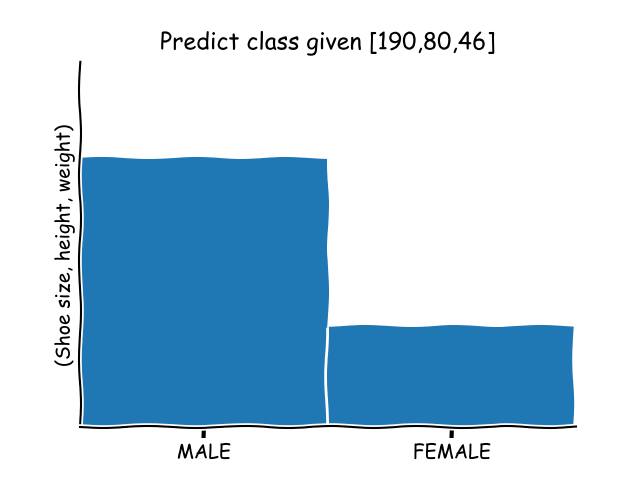Machine Learning Classifiers can be used to predict. Given example data (measurements), the algorithm can predict the class the data belongs to.

Start with training data. Training data is fed to the classification algorithm. After training the classification algorithm (the fitting function), you can make predictions.

Related course:

## Machine Learning Classification

In the example below we predict if it’s a male or female given vector data.

We start with training data. In this example we have a set of vectors (height, weight, shoe size) and the class this vector belongs to:

Define a vector for your prediction in the same format (height, weight, size). If you want, you can also get this from console input:

Then we fit the training data and predict in this style:That gives us this code: